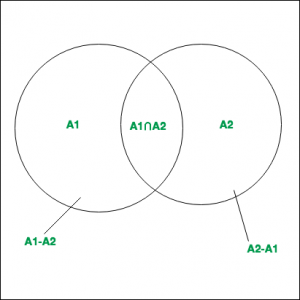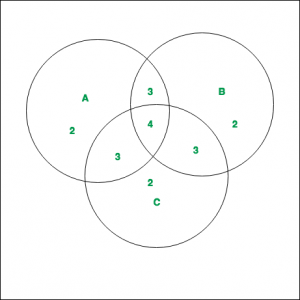# Inclusion-Exclusion and its various Applications

• Difficulty Level : Easy
• Last Updated : 17 Aug, 2021

In the field of Combinatorics, it is a counting method used to compute the cardinality of the union set. According to basic Inclusion-Exclusion principle

• For 2 finite setsand, which are subsets of Universal set, thenandare disjoint sets.• Hence it can be said that,.

• Similarly for 3 finite setsand## Principle :

Inclusion-Exclusion principle says that for any number of finite sets, Union of the sets is given by = Sum of sizes of all single sets – Sum of all 2-set intersections + Sum of all the 3-set intersections – Sum of all 4-set intersections .. +Sum of all the i-set intersections.

In general it can be said that,Properties :

1. Computes the total number of elements that satisfy at least one of several properties.
2. It prevents the problem of double counting.

Example 1:
As shown in the diagram, 3 finite sets A, B and C with their corresponding values are given. ComputeSolution :
The values of the corresponding regions, as can be noted from the diagram are –By applying Inclusion-Exclusion principle,#### Applications :

• Derangements
To determine the number of derangements( or permutations) of n objects such that no object is in its original position (like Hat-check problem).
As an example we can consider the derangements of the number in the following cases:
For i = 1, the total number of derangements is 0.
For i = 2, the total number of derangements is 1. This isFor i = 3, the total number of derangements is 2. These areand 3 1 2.

My Personal Notes arrow_drop_up
Recommended Articles
Page :